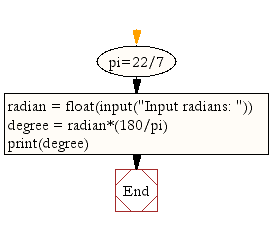﻿ Python Math: Convert radian to degree - w3resource# Python Math: Convert radian to degree

## Python Math: Exercise-2 with Solution

Write a Python program to convert radian to degree.
Note: The radian is the standard unit of angular measure, used in many areas of mathematics. An angle's measurement in radians is numerically equal to the length of a corresponding arc of a unit circle; one radian is just under 57.3 degrees (when the arc length is equal to the radius).

Sample Solution:-

Python Code:

``````pi=22/7
print(degree)
```
```

Sample Output:

```Input radians: 10
572.7272727272727
```

Pictorial Presentation:Flowchart:## Visualize Python code execution:

The following tool visualize what the computer is doing step-by-step as it executes the said program:

Python Code Editor:

Have another way to solve this solution? Contribute your code (and comments) through Disqus.

What is the difficulty level of this exercise?

Test your Programming skills with w3resource's quiz.

﻿

```>>> students = [{'name': 'John', 'score': 98}, {'name': 'Mike', 'score': 94}, {'name': 'Jennifer', 'score': 99}]